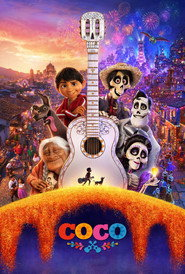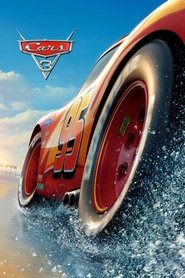type to search

Disney and Pixar Movies - Page 2

:) :) :) :) :) :) :) :)))))))))))))))))))))))))))))))))))))))))))))))))))))))))))))))))))))))))))))))
96 users · 198 views

avg. score: 38 of 55 (69%)
required scores: 1, 30, 37, 45, 51

How many have you seen?

Page 2 of 2
41Tangled (2010)
42Tarzan (1999)
43Toy Story (1995)
44Toy Story 2 (1999)
45Toy Story 3 (2010)
46Up (2009)
47WALL·E (2008)
48Wreck-It Ralph (2012)
49Inside Out (2015)
50Finding Dory (2016)
51Coco (2017)
52Cars 3 (2017)
53The Good Dinosaur (2015)
54Sleeping Beauty (1959)
55Brother Bear 2 (2006)
Page 2 of 2Your Results
Click the SHOW MY RESULTS button
above to calculate your results.
Click movies you've seen